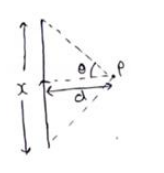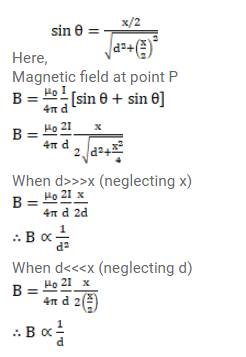# Consider a straight piece of length x of a wire carrying a current i.

Question:

Consider a straight piece of length $x$ of a wire carrying a current i. Let $P$ be a point on the perpendicular bisector of the piece, situated at a distance $d$ from its middle point. Show that for $d>>x$, the magnetic field at $P$ varies as $1 / d^{2}$ whereas for $d<<$, it varies as $1 / d$.

Solution: